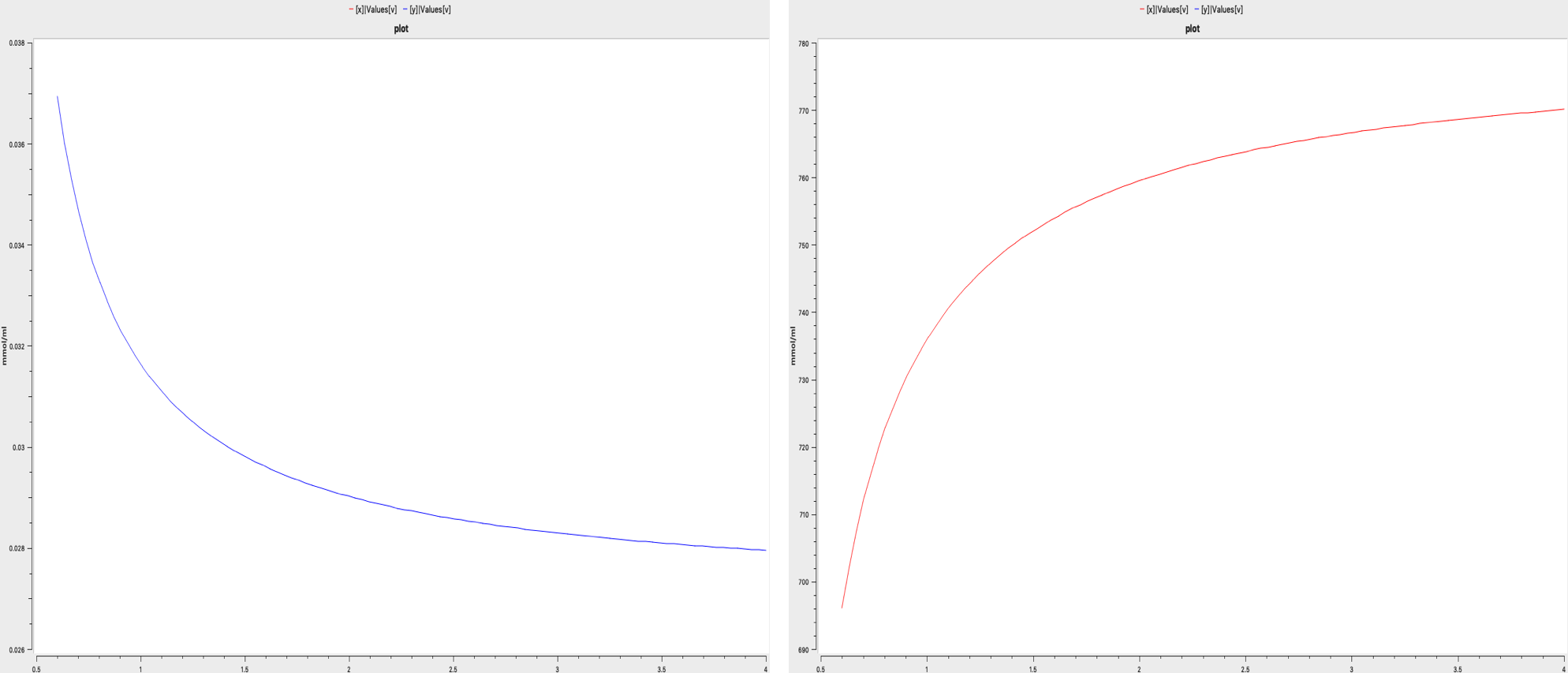## Ledzewicz2013 - On optimal chemotherapy with a strongly targeted agent for a model of tumor immune system interactions with generalized logistic growthModel Identifier
BIOMD0000000919
Short description
On optimal chemotherapy with a strongly targeted agent for a model of tumor-immune system interactions with generalized logistic growth.
Ledzewicz U1, Olumoye O, Schättler H.
1
Dept. of Mathematics and Statistics, Southern Illinois University Edwardsville, Edwardsville, Illinois 62026-1653, USA. uledzew@siue.edu
Abstract
In this paper, a mathematical model for chemotherapy that takes tumor immune-system interactions into account is considered for a strongly targeted agent. We use a classical model originally formulated by Stepanova, but replace exponential tumor growth with a generalised logistic growth model function depending on a parameter v. This growth function interpolates between a Gompertzian model (in the limit v → 0) and an exponential model (in the limit v → ∞). The dynamics is multi-stable and equilibria and their stability will be investigated depending on the parameter v. Except for small values of v, the system has both an asymptotically stable microscopic (benign) equilibrium point and an asymptotically stable macroscopic (malignant) equilibrium point. The corresponding regions of attraction are separated by the stable manifold of a saddle. The optimal control problem of moving an initial condition that lies in the malignant region into the benign region is formulated and the structure of optimal singular controls is determined
Format
SBML (L2V4)
Related Publication
• On optimal chemotherapy with a strongly targeted agent for a model of tumor-immune system interactions with generalized logistic growth.• Ledzewicz U, Olumoye O, Schättler H
• Mathematical biosciences and engineering : MBE , 6/ 2013 , Volume 10 , Issue 3 , pages: 787-802 , PubMed ID: 23906150
• Dept. of Mathematics and Statistics, Southern Illinois University Edwardsville, Edwardsville, Illinois 62026-1653, USA. uledzew@siue.edu
• In this paper, a mathematical model for chemotherapy that takes tumor immune-system interactions into account is considered for a strongly targeted agent. We use a classical model originally formulated by Stepanova, but replace exponential tumor growth with a generalised logistic growth model function depending on a parameter v. This growth function interpolates between a Gompertzian model (in the limit v → 0) and an exponential model (in the limit v → ∞). The dynamics is multi-stable and equilibria and their stability will be investigated depending on the parameter v. Except for small values of v, the system has both an asymptotically stable microscopic (benign) equilibrium point and an asymptotically stable macroscopic (malignant) equilibrium point. The corresponding regions of attraction are separated by the stable manifold of a saddle. The optimal control problem of moving an initial condition that lies in the malignant region into the benign region is formulated and the structure of optimal singular controls is determined.
Contributors
Submitter of the first revision: Mohammad Umer Sharif Shohan
Submitter of this revision: Mohammad Umer Sharif Shohan

is (2 statements)
BioModels Database MODEL2003060003
BioModels Database BIOMD0000000919

isDescribedBy (1 statement)
PubMed 23906150

hasTaxon (1 statement)
Taxonomy Homo sapiens

isInstanceOf (2 statements)
Mathematical Modelling Ontology Ordinary differential equation model
Taxonomy Homo sapiens

hasProperty (1 statement)
Mathematical Modelling Ontology Ordinary differential equation model

Curation status
Curated

Modelling approach(es)

Tags

#### Connected external resources

SBGN view in Newt Editor

Name Description Size Actions

### Model files

Ledzewicz2013.xml SBML L2V4 representation of Ledzewicz2013 - On optimal chemotherapy with a strongly targeted agent for a model of tumor immune system interactions with generalized logistic growth 31.82 KB Preview | Download

Ledzewicz2013.cps COPASI version 4.27 (Build 197) representation of Ledzewicz2013 - On optimal chemotherapy with a strongly targeted agent for a model of tumor immune system interactions with generalized logistic growth 63.84 KB Preview | Download
Ledzewicz2013.sedml SEDML L1V2 representation of Ledzewicz2013 - On optimal chemotherapy with a strongly targeted agent for a model of tumor immune system interactions with generalized logistic growth 5.70 KB Preview | Download
• Model originally submitted by : Mohammad Umer Sharif Shohan
• Submitted: Mar 6, 2020 6:10:58 PM
##### Revisions
Legends
: Variable used inside SBML models

Species
Species Initial Concentration/Amount
y 0.1 mmol
x

Tumor Volume
600.0 mmol
Reactions
Reactions Rate Parameters
=> y compartment*alpha alpha = 0.1181
=> y; x compartment*mu_I*(1-beta*x)*x*y beta = 0.00264; mu_I = 0.00484
y => compartment*delta*y delta = 0.37451
x => ; y compartment*gamma*x*y gamma = 1.0
=> x compartment*mu_C*x*(1-(x/x_inf)^v) v = 1.0; mu_C = 0.5599; x_inf = 780.0Curator's comment:
(added: 06 Mar 2020, 18:10:35, updated: 06 Mar 2020, 18:10:35)
The model has been encoded using COPASI 4.24 (Build 197) and the figure 1 of the publication has been reproduced using COPASI. The model has the initial value for v as 0.6 not 0.5 as it seems there are some noises when 0.5 is used as the value of v. The figure looks exactly as the one present in the figure of the publication